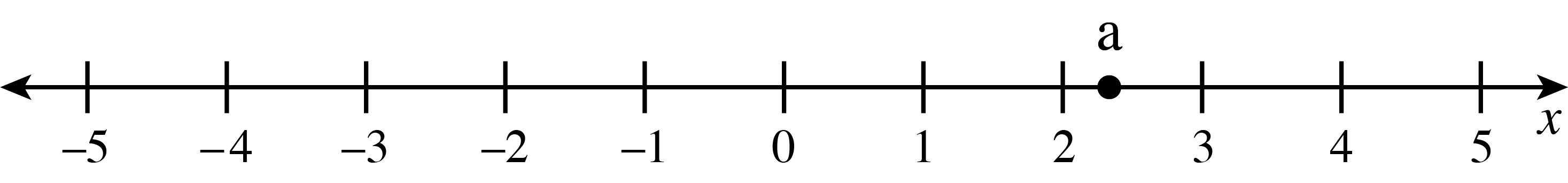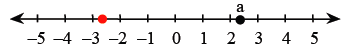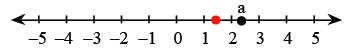### Home > CC3MN > Chapter 1 > Lesson 1.1.3 > Problem1-23

1-23.

Copy the number line below onto your paper. Locate the following numbers by placing the lowercase letters a through f on the number line corresponding to the values given below. Part (a) is done for you.1. $2\ \frac{1}{3}$

1. $−2.7$

Is $−2.7$ closer to $−2$ or $−3$?1. $0.9−0.04$

1. $-0.2$

Determine on which side of zero the point is located.

1. $33\frac{1}{3}\%$ of $12$

Change the percent to a fraction. Then, multiply the fraction by $12$.

1. $\frac{7}{10}+\frac{2}{3}$